## Plus Blog

September 6, 2010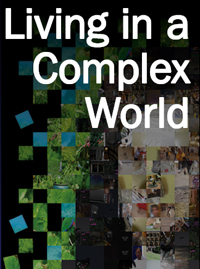Many things in life are more than the sum of their parts. Whether its the behaviour of crowds of people, flocking birds or shoaling fish, the unpredictable patterns of the weather or the complex structure of the Internet, it's often the interaction between things, rather than the things themselves, that generates complexity.

It's a challenge to science, whose traditional approach of taking things apart and looking at the individual bits doesn't work when faced with emergent complexity. But there are mathematical techniques to understand this phenomenon. The Living in a Complex World website, originally launched to accompany an exhibit at the Royal Society Summer Science exhibition, explores complexity in the real world and has some great factsheets looking at the maths used to understand it. It's well worth a look!

August 27, 2010

Here are some pictures from the ICM 2010: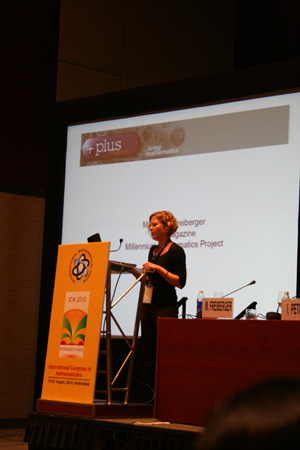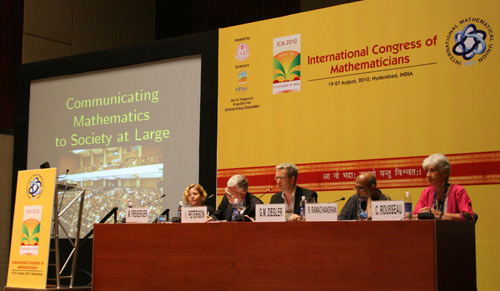Well, maybe not quite ... it's a panel discussion on popularisation of maths. (Thanks to Jaime Carvalho e Silva for both of these photos.)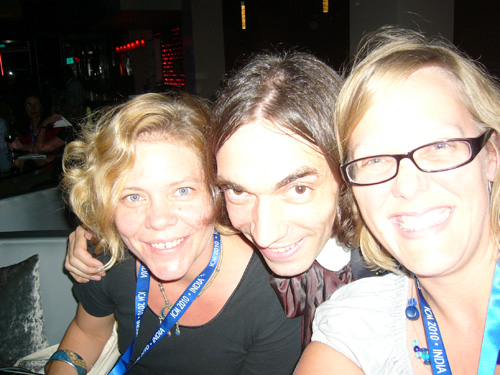Plus with Cédric Villani.3000 mathematicians trying to have dinner.3000 mathematicians trying to have lunch.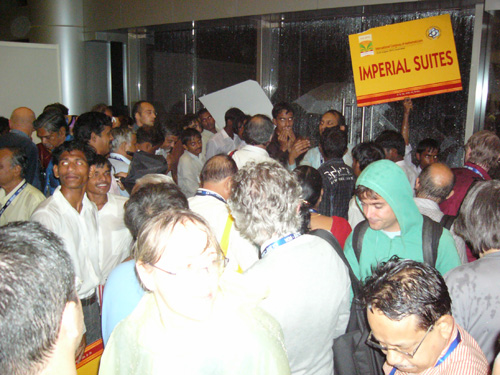3000 mathematicians trying to catch a hotel bus.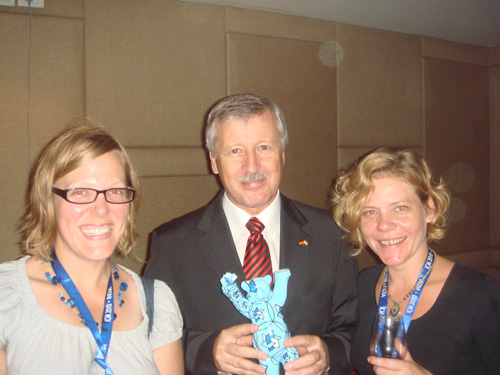Plus with Christian Schlaga, Germany's acting ambassador to India. It's a long story, but basically Plus ended up with a sculpture of the Berlin bear (with a maths design) that had been presented to Schlaga at the German embassy's reception at the ICM.August 20, 2010

What would you think if the nice café latte in your cup suddenly separated itself out into one half containing just milk and the other containing just coffee? Probably that you, or the world, have just gone crazy. There is, perhaps, a theoretical chance that after stirring the coffee all the swirling atoms in your cup just happen to find themselves in the right place for this to occur, but this chance is astronomically small.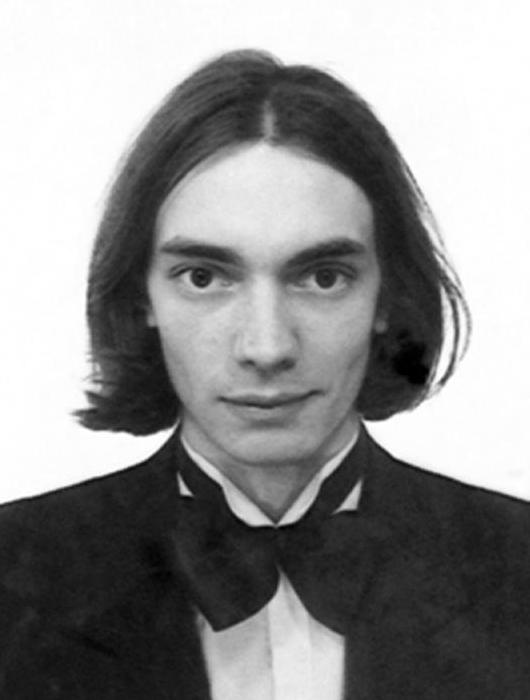Cédric Villani, Institut Henri Poincaré
Fields medallist 2010.

The fact that such spontaneous separation never occurs in practice is an illustration of a deep physical law: it says that the entropy of a system, a measure of its complexity, almost always increases as time passes. When you first pour the milk into your coffee, for a split second milk and coffee will be neatly separated, but soon the milk disperses. The mixture of milk and coffee becomes more and more complex, until it reaches an equilibrium when both are completely mixed up and complexity is at its peak.

In the late 19th century the physicist Ludwig Boltzmann studied this phenomenon, looking at what happens when a gas is released into a room from a bottle. He came up with an equation describing the evolution of this process — the change over time — in terms of how individual atoms collide and other forces acting on the gas. His calculations showed that indeed entropy doesn't decrease. The atoms of gas start out in an ordered state — all sitting in the bottle — and end up in a state of maximum complexity, dispersed throughout the room.

Intriguingly, this result meant that there is what physicists call an arrow of time, something that isn't inherent in classical Newtonian physics. If someone showed you a movie of a billiard ball rolling across the table, then you wouldn't be able to tell if the movie was being played forwards or backwards: it's just as likely that the ball rolls one way as it is to roll in the opposite direction. If, however, someone showed you a movie of a coloured gas dispersed in a room suddenly entering a bottle, you'd know that something's wrong. The movie is being played backwards. Since the interaction of individual atoms was described in terms of Newtonian laws (which don't have a preferred direction of time), this emergence of an arrow of time created some headache for physicists. Debate on the arrow of time issue continues to this day. As the mathematician John von Neumann once put it, "nobody knows what entropy really is, so in a debate you will always have the advantage".

Villani, however, does understand was entropy is. One question that has until recently remained open was how fast the entropy of a system increases. Cédric Villani received his Fields Medal for developing rigorous mathematical techniques that provide an answer. They show that while entropy never decreases, it sometimes increases faster and sometimes slower. Based on this work, Villani developed a general theory, hypercoercivity, which applies to a broad set of situations.

Villani also used his understanding of entropy to explain a phenomenon that had puzzled physicists for 60 years. Back in the 1940s, the Soviet physicist Lev Davidovich Landau claimed that plasma, a form of matter similar to gas, spreads and reaches its equilibrium state without increasing its entropy. Landau argued that unlike gas, whose approach to equilibrium is driven by particle collisions (which also lead to a loss of order), plasma reaches equilibrium through a decay in its electric field. Landau made some progress on proving his claim, but despite hard work by many physicists over 60 years, it wasn't until Villani's arrival on the scene that Landau was proved right.

All this might sound somewhat esoteric, but Villani's deep understanding of entropy has direct consequences for real-life problems. In the 18th century the French mathematician Gaspard Monge started to think about how to transport goods to various places in an efficient way. For example, you might want to distribute a bunch of letters sitting in a post office to the various addresses in a way that minimises transport cost. Villani and his colleague Felix Otto made a crucial connection between this problem and gas diffusion, by drawing the following analogy: loosely speaking, the initial state (all letters in the post office) corresponds to the ordered state of gas sitting in a bottle, while the end state (letters delivered) corresponds to a state where the gas has dispersed. To any configuration of dispersed gas particles you can assign a cost by seeing how far the particles had to travel from the original ordered state. Using this analogy and their understanding of entropy, Villani and Otto made important contributions to optimal transport theory.

So next time you have a cup of milky coffee or receive a letter, stop to think that a deep understanding of either, or even better a combination of both, could have earned you a Fields medal.

You can find out more about Villani's work in this excellent description on the ICM website, on which this blog post was based. To find out more about the Boltzmann equation and optimal transport problems, read the Plus article Universal Pictures.

August 20, 2010

Suppose you throw an equal number of white and black balls into a rectangular box which is, say, 30 balls long, 10 balls wide and is now 5 layers deep in balls. What it the probability that you have a run of touching white balls from one end of the box to the other?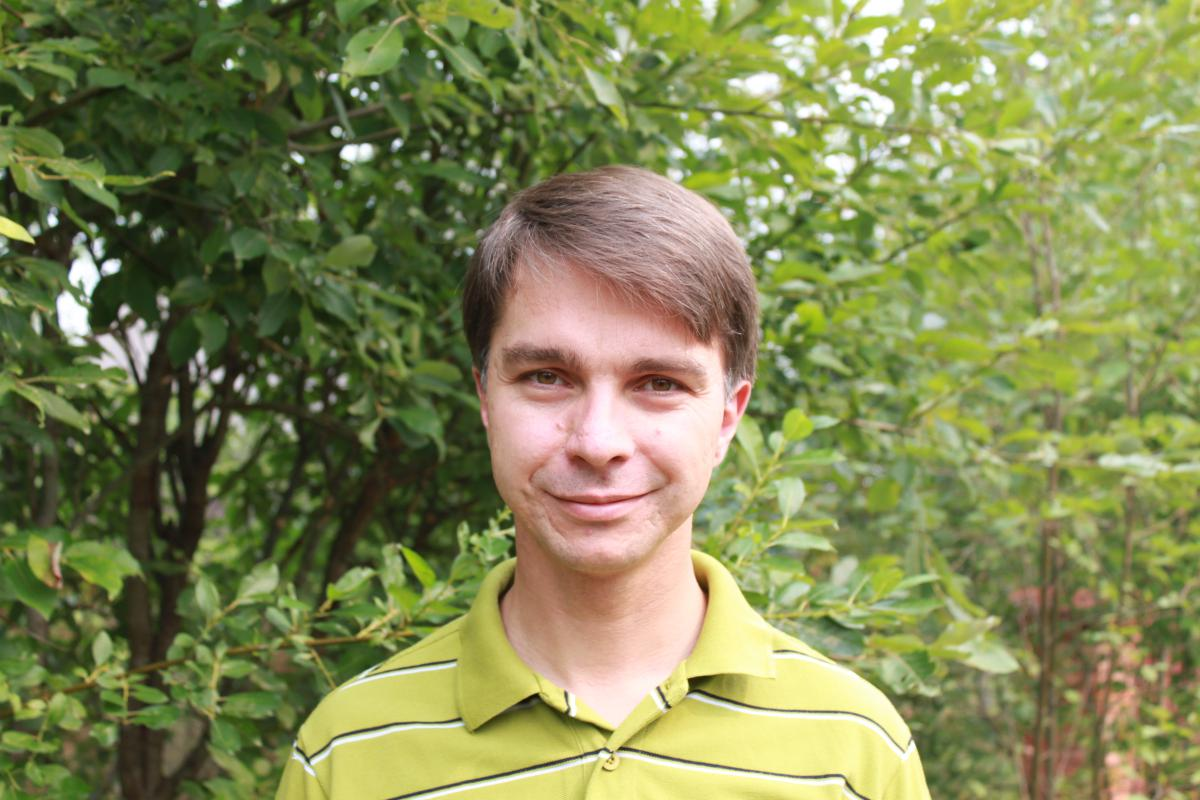Stanislav Smirnov

This question, asked all the way back in 1894 in the first issue of the American Mathematical Monthly, turned out be far from simple. In fact it appears to be the earliest reference to the rich mathematical field of percolation theory, according to Harry Keston, who told the International Congress of Mathematicians about Stanislav Smirnov's work in this area that lead to Smirnov winning the 2010 Fields Medal.

Just as the name conjures up the image of water percolating through soil or porous rock, percolation theory models this mathematically as a liquid flowing through a lattice of pipes. The points where the pipes join (mathematically known as the vertices of the lattice) are either blocked, stopping the flow of liquid, or open, allowing the liquid to flow through. You can imagine that if each vertex has a high probability p of being open (and the lattice is very porous like sand) then we can be fairly certain that the liquid will flow through the lattice of pipes. And if the probability p that each vertex is open is low (and the lattice is impermeable like hard clay) then we can be fairly sure the liquid is going to get stuck and not make it the whole way through.

It turns out that there is a particular critical probability for the vertices being open, pc, that determines exactly when a liquid can percolate across the lattice. If the probability that the vertices are open is below this critical probability, p < pc, then the liquid will never percolate through the lattice. When the probability of the vertices being open passes this critical point, and p > pc, the system flips behaviour and the liquid can trickle all the way through. (You can read an excellent more technical introduction in Percolation: Slipping through the cracks.)

Physicists are interested in this area as it is one of the simplest models to have a phase transition, where the behaviour of the system flips at a certain critical point. Phase transitions are an important part of physics, for example understanding the change between different phases of matter. Water immediately starts to boil once it is above a certain critical temperature (just under 100 °C at sea level) but will not boil at lower temperatures. What is particularly interesting is what happens at these critical points. How likely is it for the liquid to percolate across the lattice, called the crossing probability, when the vertices are open with the exact probability p = pc?

But the systems physicists are interested aren't neatly space lattices in regular shapes. Instead physicists are interested in what happens at the smallest possible scale when the spacing in the mesh of the lattice becomes finer and finer, which they call taking the scaling limit. For a given mesh size it is possible to calculate the crossing probability, and physicists were convinced from physical evidence that the crossing probability existed for the scaling limit. That is, they thought that as the mesh got finer and finer the crossing probabilities would get closer and closer to a final value that would be the crossing probability for the scaling limit. And the physicist John Cardy was even able to give a formula for calculating this final value. However, no one was able to prove mathematically either that this value would exist, or that the formula was correct.

In 2001 physicists and mathematicians alike breathed a sigh of relief when Smirnov proved that the crossing probability existed for the scaling limit for a two-dimensional triangular lattice, and that it was equal to the value calculated by Cardy's formula. Keston, himself a pioneer in the percolation theory, said that Smirnov's work would make statistical physicists very happy as it confirmed their assumptions and put the area on a solid mathematical foundation. And it is hoped that the novel techniques Smirnov used to prove this and related results will allow him and others to extend these results to any two-dimensional lattice (square, hexagonal, and so on), proving that Cardy's conjecture is universal and independent of the lattice being used.

At the end of Shmirnov's own presentation of his work at the ICM, he was asked if his work could be extended to three-dimensional lattices. Smirnov held up his hands, very little is known about how these models work in three-dimensions. Perhaps Smirnov, or a future Fields medallist, will take us there.

August 19, 2010

Elon Lindenstrauss got the Fields Medal for developing tools in the area of dynamical systems and using them to crack hard problems in number theory.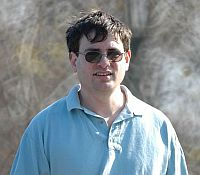Elon Lindenstrauss, Princeton University
Fields medallist 2010.

As the name suggests, number theory studies the basic properties of numbers. The whole numbers 1, 2, 3, etc are probably the first thing that spring to mind when you think about numbers. Close to follow are the rational numbers: these are the fractions, numbers of the form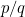, where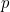and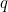are both whole numbers. But there also irrational numbers, which can't be written as fractions. An example is the number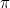: some people write it as 22/7, but that's just an approximation: it's close to, but not exactly equal to it. In fact, there isn't any fraction that's exactly equal to.

In turns out that you can approximate an irrational number, call it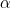, by a fraction to any degree of accuracy. If you give me a really small number, then no matter how smallis, I can find you a fraction that's withinof. But some approximations are better than others. The fraction 2147865/68341 is a tiny bit closer tothan 22/7, but it's also much more horrible to write down because it has such a large denominator (and as a result a very large numerator). So what's the ideal relationship between the accuracy of approximation and the denominator of a fraction?

In the 19th century the German mathematician Johan Dirichilet came up with a notion of this ideal relationship. He decided that an approximationof an irrational numbershould be no further fromthan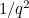. In other words, if the denominatoris large, (so that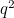is even larger and thereforevery small), then the fraction should make up for this by being close enough (within) of. Dirichilet proved, and the proof wasn't very hard, that given any irrational number, you can always find infinitely many fractionswhich satisfy this criterion. So there's a "nice" approximation, in Dirichilet's sense, for any level of accuracy.

It turns out that something similar is true for pairs of irrational numbersand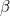. There are infinitely many fractionsand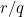which are nice simultaneous approximations ofand: the difference betweenandtimes the difference betweenandis less than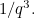Put in the form of an equation, this is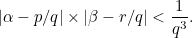Since pairs of numbers can be interpreted as the coordinates of a point on a 2D plane, this result gives a measure of how well points with irrational coordinates can be approximated using points with rational coordinates that have the same denominator.

In the twentieth century the mathematician John Littlewood decided that we should be able to do even better than this. Given any two irrational numbersandand anthat's as small as you like, there should be fractionsandso that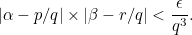The statement seemed like an easy generalisation, but no-one has so far been able to prove it. It's become known as the Littlewood conjecture.

Number theory is littered with statements that look like they should be easy to prove but turn out to be incredibly hard. In these cases you have to look for clever tools to help you find a solution. In his work Elon Lindenstrauss did just that, using tools from dynamical systems theory. As an example of a dynamical system, think of the 2D plane in which every point is defined by its co-ordinates, a pair of numbers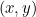. Now take any such pointand shift it by a certain distanceto the right and up by another distance. This rule gives you a dynamical system. You can apply it again and again and see what happens to the trajectories of various points.

In the case of the plane, nothing very interesting happens, as trajectories just move further and further away from the centre of the plane, given by the coordinates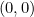. If, however, if you look at the surface of a doughnut, things get more interesting. You can make such a surface by taking a square from the plane, turning it into a cylinder by gluing together the left and right edges, and then bending it around and gluing together the circles on either end of the cylinder. In this way, the doughnut's surface inherits the coordinates defined on the original square. Things now become more interesting as you shift points around as before, using the numbersand. Trajectories can travel round and round and visit the same patch of doughnut lots of times.

It turns out that if your two numbersandare irrational, then the dynamical system is what's called ergodic: loosely speaking, trajectories will visit every patch of the doughnut surface and patches of equal area will see comparable rates of traffic. And here is the connection with the Littlewood conjecture: suppose that the pair of numbersand, the distances by which you're shifting points, are the pair of irrational numbers you're trying to simultaneous approximate by fractions. It turns out that proving the Littlewood conjecture is equivalent to showing that you can get every pointsufficiently close to the point, just by shifting along using the numbersanda suitable number of times. The number of times you need to shift along gives you the denominatoryou're after.

Using a more complicated dynamical system, Lindenstrauss and his colleagues made massive progress towards a proof of the elusive Littlewood conjecture. They showed that if there are any pairs of numbers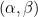that can't be approximated in the nice way stipulated by the conjecture, then they make up only a negligible portion of the plane in which they live. There are pairs for which the conjecture isn't yet proven, in fact there's infinitely many of them, but as Lindenstrauss showed, collectively they are nothing more than drops in the ocean of the 2D plane.

It's this progress on Littlewood's conjecture that forms part of the body of work for which Lindenstrauss is being honoured. You can find out more about his work in this excellent description on the ICM website.

August 19, 2010

Results in mathematics come in several flavours — theorems are the big important results, conjectures will be important results one day when they are proved, and lemmas are small results that are just stepping stones on the way to the big stuff. Right? Then why has the Fields medal just been awarded to Ngô Bào Châu for his proof of a lemma?It turn's out that Ngô's lemma, formulated in 1970 as part of the famous Langlands programme, wasn't so small after all. And after an enormous amount of mathematical theory came to rely on this unproven lemma, it got a promotion and became known as the Fundamental Lemma.

In the 1970's the mathematician Robert Langland had a grand vision that could bring together the seemingly unrelated fields of group theory, number theory, representation theory and algebraic geometry. Langlands work laid out a mathematical map connecting these diverse areas of mathematics which has lead to a large area of research known as the Langlands program. One of the most important tools in this work is the trace formula, an equation which allows arithmetic information to be calculated from geometric information, itself linking together the disparate concepts of the continuous (a property of things that can be divided into infinitisemal parts, which include geometric objects such as lines, surfaces and the the three-dimensional space we live in) and the discrete (describing things that come in whole indivisible parts, such as the whole numbers which are studied in number theory).

However in order for the trace formula to be applied in any useful way in the Langlands program, an apparently relatively simple condition, that two complicated sums were equal, needed to hold. Langlands and others assumed this condition was true and stated it as a lemma, and many results in the Langlands programme relied on this lemma being true. Langlands set a graduate student the exercise of proving this lemma, but when the student, and then many others failed to prove the result was true, it became known as the Fundamental Lemma. And it's unproven state became a thorn in the side of the Langlands programme.

Finally the thorn has been removed as Ngô has proved the Fundamental Lemma using some surprising methods. Unexpectedly he was able to use geometric objects, called Hitchin fibrations, solve this problem in pure mathematics. And not only did he prove this result, he provided a deeper understanding of this area of mathematics. Ngô not only revealed the the mathematical iceberg of which the tip was the Fundamental Lemma, he also provided a way to understand the whole ice field, said James Arthur, who explained Ngô's acheivements to the International Congress of Mathematicians after Ngô received his Fields Medal.

Ngô's work highlights the unexpected nature of mathematics, how even the smallest steps in a proof can turn out to be giant leaps in knowledge and understanding. And Ngô's career as an explorer in the mathematical wilderness has only just begun, and we all look forward to the new frontiers he will go on to discover.

You can read more about Ngô Bào Châu's work on the ICM website. And you can read more about group theory, number theory and algebraic geometry on Plus.